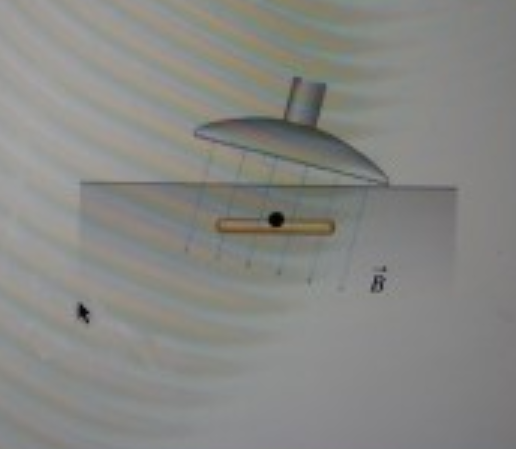# Problem: A metal detector uses a changing magnetic field to detect metallic objects. Suppose a metal detector that generates a uniform magnetic field perpendicular to its surface is held stationary at an angle of 15.0° to the ground, while just below the surface there lies a silver bracelet consisting of 6 circular loops of radius 5.00 cm with the plane of the loops parallel to the ground. If the magnetic field increases at a constant rate of 0.0250 T/s,1. what is the induced emf ε? Take the magnetic flux through an area to be positive when B crosses the area from top to bottom. 2. Which of the following is the reason why the magnetic flux through the bracelet is changing? A) The bracelet is moving in the magnetic field. B) The magnetic field is changing direction with respect to the bracelet. 3. The diagram below shows a side view of the situation described in this problem. The bracelet is depicted as a single loop parallel to the ground. Draw the are vector A for the bracelet. Use the black dot as the starting point of A, that is, make certain to draw your vector so that  its tail is on the dot. Only the orientation of your vector will be graded, not its length.

###### FREE Expert Solution

Induced emf:

$\overline{){\mathbf{\epsilon }}{\mathbf{=}}{\mathbf{-}}{\mathbf{N}}{\mathbf{B}}{\mathbf{A}}{\mathbf{c}}{\mathbf{o}}{\mathbf{s}}{\mathbf{\theta }}}$

1.

A = πr2 = π(5.00 × 10-2)2 = 7.85398 × 10-3 m2

91% (266 ratings)###### Problem Details

A metal detector uses a changing magnetic field to detect metallic objects. Suppose a metal detector that generates a uniform magnetic field perpendicular to its surface is held stationary at an angle of 15.0° to the ground, while just below the surface there lies a silver bracelet consisting of 6 circular loops of radius 5.00 cm with the plane of the loops parallel to the ground. If the magnetic field increases at a constant rate of 0.0250 T/s,

1. what is the induced emf ε? Take the magnetic flux through an area to be positive when B crosses the area from top to bottom.

2. Which of the following is the reason why the magnetic flux through the bracelet is changing?

A) The bracelet is moving in the magnetic field.
B) The magnetic field is changing direction with respect to the bracelet.

3. The diagram below shows a side view of the situation described in this problem. The bracelet is depicted as a single loop parallel to the ground. Draw the are vector A for the bracelet. Use the black dot as the starting point of A, that is, make certain to draw your vector so that  its tail is on the dot. Only the orientation of your vector will be graded, not its length.